American Journal of Modern Physics
Volume 4, Issue 2-1, April 2015, Pages: 15-18

Relativity of time and instantaneous interaction of charged particles

Wolfgang Engelhardt

Retired from Max-Planck-Institut für Plasmaphysik, D-85741Garching, Germany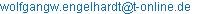Wolfgang Engelhardt. Relativity of Time and Instantaneous Interaction of Charged Particles. American Journal of Modern Physics. Vol. 4, No. 2-1, 2015, pp. 15-18. doi: 10.11648/j.ajmp.s.2015040201.13

Abstract: The interaction between charged particles through quasi-static fields must occur instantaneously; otherwise a violation of the energy principle would occur. As a consequence, the instantaneous transmission of both energy and information over macroscopic distances is feasible by using the quasi-static fields which are predicted by Maxwell’s equations. This finding is incompatible with the «relative simultaneity» following from the time transformation postulated by the special theory of relativity.

Keywords: Classical electrodynamics, Quasi-static electromagnetic fields, Transmission of information

1. Introduction

In 1887 Woldemar Voigt  postulated the homogeneous wave equation to be form-invariant against transformation into a moving reference system. This required making Newton’s absolute time a function of space with the consequence that the propagation velocity of waves as measured by an observer became constant, independent of the observer’s velocity. The idea was ignored in acoustics, as it obviously contradicts known facts, but it was taken seriously in case of light by Poincaré  who multiplied Voigt’s formulae by a constant factor in order to symmetrize the transformation with its inverse. He named the result after Lorentz. The underlying assumption c = const seemed to be supported by the generalized relativity principle which Poincaré thought to be a law of nature.

Although Lorentz’s own transformation  was different from Voigt’s or Poincaré’s, it had in common a curious transformation of time which Einstein  supposed to hold in general, not only in the context of light wave propagation. Like Poincaré Einstein cherished the relativity principle and derived the «Lorentz Transformation» similarly as Voigt from the postulate c = const. As he did not cite anybody, we do not know whether he was aware of Voigt’s paper. It is a fact , however, that he did not use Lorentz’s or Poincaré’s nomenclature, but adopted Voigt’s.

The new relativity of simultaneity as imposed by the Lorentz transformation required that energy or information could not propagate faster than light; otherwise causality would be violated between systems moving at constant velocity relative to each other. On the other hand, there are good reasons both experimentally and theoretically to assume that it is possible to transmit information faster than light [6 - 9]. It is very obvious: Either one admits the validity of the Lorentz transformation or alternatively the possibility of instantaneous transmission of energy. A compromise is hardly possible, as both conjectures are not compatible with each other. Since the Lorentz transformation has already been refuted in first order of v/c by the Sagnac effect  and by lacking stellar aberration in spectroscopic binary systems , one is compelled to return to the Newtonian concept of absolute time which allows a clear definition of simultaneity.

In this paper we add further evidence for the instantaneous transmission of energy between charged particles or current loops. Our argument is based on Maxwell’s equations and on the requirement of conserving energy in the interaction process. In Sec. 2 we reformulate Maxwell’s equations in order to clarify that they predict not only wave fields, but also instantaneous, quasi-static fields that do not spread at finite velocity. It is well known that these fields, which decay rapidly with distance from the source, are capable of transmitting energy.  In the remaining Sections we discuss in detail examples of instantaneous energy transfer between charged bodies or current loops.

2. Quasi-static Fields as Predicted by Maxwell’S Equations

Due to the linearity of Maxwell’s equations the fields may be split up into quasi-static, instantaneous contributions, and into wave parts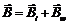,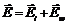. In case of the electric field the instantaneous part consists of the irrotational Coulomb field and an induced rotational field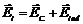. The wave fields obey inhomogeneous hyperbolic equations: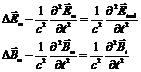(1)

where we have used, e.g.: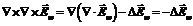.

The instantaneous fields appear as sources in (1) and can be calculated from a given charge and current distribution: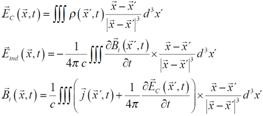(2)

The set of equations (1, 2) is entirely equivalent to Maxwell’s equations which may be checked by insertion into the first order system . In the present formulation it becomes obvious that Maxwell’s theory predicts not only wave propagation at the velocity of light (1), but also instantaneous fields as described by the quasi-static integrals (2). A complete cancellation of the two kinds of fields is not possible, as was pointed out in  and is obvious from (1): If the sum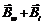, e.g., would vanish for a certain interval of time, one would have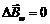so that the wave fieldwould vanish everywhere when the usual boundary condition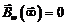is imposed.

Note that the fields (2) are typically dipole fields which decay off proportional to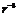. When they are used for energy transfer, the range of interaction is much shorter than in case of wave fields. In practice they are taken as instantaneous anyway in technical applications, but this is not just an approximation, but follows exactly from (2).

At this point we will neither discuss whether the formal integrals in (2) always exist, nor whether the inhomogeneous wave equations (1) do have solutions in general. This matter has been dealt with in . Here we will produce an energy argument in Sec. 3 which demonstrates independently of the subtleties of Maxwell’s equations, that a delayed Coulomb interaction between charged particles would lead to a clash with the energy principle. Similar considerations apply to the magnetic interaction in technical transformers (Sec. 4).

3. Interaction of two Charges Coupled by the Coulomb Field

Let us consider two positive charges which are placed at a distance R. In Fig. 1 charge B is rigidly attached to a heavy wall, whereas charge A can be moved by a mechanical force along a distance r. When charge A is pushed against B, a force must be applied against the repulsive electric force, and a certain amount of potential energy is invested. Since charge A moves in a conservative potential which does not vary in time, the invested work is recovered when the charge returns to its initial position.Figure 1. Interaction of two charges (charge B rigidly attached)

Next we consider Fig. 2 where charge B is attached to a flexible spring. Initially the spring is somewhat compressed due to the repulsive force between the charges.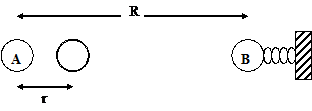Figure 2. Interaction of two charges (charge B elastically attached)

When charge A is pushed against B, the spring is compressed even more, and, because of its inertia, charge B will oscillate after charge A has returned to its initial position. Obviously, energy has been transmitted from A to B through the quasi-static electric field. The oscillation energy must have been supplied by the mechanical force acting on charge A. This is easily explained: Charge A was moving in a conservative potential which was, however, not constant in time, since charge B was yielding to the increased force due to its elastic fixture. If one carries out the exact calculation assuming that the charges were coupled by the Coulomb force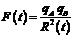(3)

one finds, of course, that the energy appearing in the oscillation of charge B was exactly supplied by the mechanical force which moved charge A.

In this seemingly trivial example we have assumed in (3) that both charges are coupled instantaneously. Let us now assume that a time R/c elapses before charge B can "realize" that charge A is moving. If the cyclic motion of charge A is completed within a short time τ < R/c, charge B cannot react during the cycle so that charge A still moves in a constant conservative potential like in Fig. 1. The mechanical force does not produce any total work during the cycle, but after a delay time R/c charge B will start to oscillate. Its energy comes out of nothing as a consequence of our assumption that the action of charge A on B is delayed. As long as we believe in the conservation of energy we must conclude that the coupling is determined by (3), and the energy transfer occurred instantaneously.

One might argue that the acceleration of charge A produced a wave containing energy which was transported to B at finite velocity. It is, of course, true that the acceleration of charges produces waves according to Maxwell’s theory as described by (1). This holds, however, in both cases of Fig. 1 and Fig. 2. It is entirely independent of charge B being rigidly or elastically attached to the wall. In both cases the mechanical force must supply a small amount of energy which is carried away by the wave and must be accounted for in the energy balance. The salient point is, however, that the energy balance completed at charge A during a cycle cannot depend on charge B oscillating in the future or not. There must be an instantaneous feedback from charge B to charge A in order to balance correctly the work at charge A with any oscillation energy produced at charge B. This necessary feedback is provided by the Coulomb force (3), or equation (2), in general.

Furthermore, it should be noted that the wave travels perpendicular to the acceleration of charge A and does not reach charge B at all, as it is placed in line with the acceleration vector. Thus, the production of waves cannot explain the missing energy source in case of Fig. 2, when delayed action is postulated.

4. Interaction of two Current Loops

All the power produced by the electric companies and transmitted to the consumers passes several times through transformers. In this Section we show that the transmission from the primary to the secondary circuits must occur instantaneously as described by Maxwell’s equations.

In principle, a transformer consists of two current loops as sketched in Fig. 3.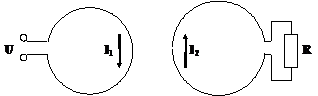Figure 3. Interaction of two current loops

Applying Faraday’s law of induction and the laws of Ampère and Ohm one has the transformer equations :(4)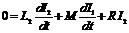(5)

where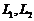are the self-inductances of the loops and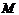is the coupling inductance. When there is no resistive load in the secondary circuit (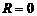), it follows from (5):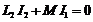for alternating currents.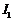and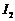are out of phase by 180 degrees. From (4) follows then that there is a phase difference of 90 degrees between the applied voltage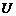and the current. The power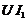injected into the primary circuit oscillates forth and back so that no energy is deposited into the (ideal) transformer on average.

If the secondary resistance is finite, a phase shift occurs which may be calculated by solving the differential equations (4) and (5). As a result the time integral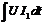does not vanish anymore, but supplies the energy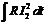which is dissipated in the secondary circuit. Energy is obviously transmitted over the distance between the loops. Since in (4, 5) Maxwell’s displacement current was neglected, the coupling of the loops was assumed to be instantaneous which holds then also for the energy transfer.

If we would assume that it takes some time for the field produced in loop 1 to travel to loop 2, induce a current there which in turn produces a magnetic field travelling back to loop 1, one would have a phase shift of more than 90 degrees between voltage and current in the primary circuit, even in the case of vanishing resistance. The integralwould not be zero on average, and energy would be lost in the ideal transformer which is supposed to contain only superconducting loops. As in the previous Section, we must conclude that the coupling in a transformer cannot be effected by an electromagnetic wave, but must be caused by quasi-static instantaneous fields, in order to avoid a clash with the energy principle. The quasi-static magnetic field can apparently be used like the quasi-static electric field to transmit information faster than light.

In industrial transformers the distance between primary and secondary circuit is chosen to be very small, but one could arrange the two loops of Fig. 3 on a common axis at an appreciable distance to measure the time of transmission. This was in fact done by Kholmetskii and co-workers . They found experimentally that a "bound" magnetic field as described by (2) is spreading at a velocity "highly exceeding the velocity of light".

5. Conclusion

In two simple examples it was demonstrated that the coupling of electric charges and currents through quasi-static fields must occur instantaneously, in order to maintain the conservation of energy. In technical applications this kind of coupling is assumed anyway, but it is frequently thought that engineers just use a practical approximation, whereas the ‘correct’ interaction requires a description in terms of travelling wave fields. Our analysis shows that this is not the case. It proves that instantaneous transmission of information over macroscopic distances is possible in agreement with Maxwell’s theory and with very recent experiments. The argument brought forward in Sec. 3 may also be applied to the mechanical force acting between the current loops of Fig.3. It could be extended to the gravitational force as well.

Having demonstrated that in classical electrodynamics energy would not be conserved, if one assumes exclusively delayed interaction by travelling wave fields, we are compelled to conclude that Maxwell’s quasi-static fields (2) are real and responsible for instantaneous interaction between electrified bodies. This fact is at variance with the relativistic postulate c = const from which the Lorentz transformation is derived. One can hardly see how this transformation can be made compatible with an infinitely fast interaction. It appears that Newton’s concept of absolute time is superior to the relativistic relative time defined in the Lorentz transformation.

References

1. W. Voigt, Ueber das Doppler´sche Princip, Nachrichten von der Königlichen Gesellschaft der Wissenschaften und der Georg–Augusts–Universität zu Göttingen, No. 2, 10. März 1887.
2. H. Poincaré, Sur la dynamique de l'électron,Rendiconti del Circolo matematico di Palermo,21 (1906) 129.
3. H. A. Lorentz, Electromagnetic phenomena in a system moving with any velocity smaller than that of light, Proceedings Acad. Sc. Amsterdam 6 (1904) 809.
4. A. Einstein, Zur Elektrodynamik bewegter Körper, Ann. d. Phys., 17 (1905) 891.
5. W. Engelhardt, On the origin of the Lorentz transformation, http://arxiv.org/abs/1303.5309
6. A. Chubykalo, A. Espinoza, R. Alvarado-Flores, A. G.. Rodriguez, Helmholtz theorem and the v-gauge in the problem of superluminal and instantaneous signals in classical electrodynamics, Foundations of Physics Letters 19 (2006) 37.
7. A.L. Kholmetskii, O.V. Missevitch, R. Smirnov-Rueda, R. Ivanov and A.E. Chubykalo, Experimental test on the applicability of the standard retardation condition to bound magnetic fields, J. Appl. Phys. 101 (2007) 023532.
8. J. H. Field, Quantum Electrodynamics and Experiment Demonstrate the Nonretarded Nature of Electrodynamical Force Fields, Physics of Particles and Nuclei Letters, 6 (2009) 320.
9. T E. Phipps, Jr., The Sherwin-Rawcliffe Experiment – Evidence for Instant Action-at-a-distance, Apeiron 16 (2009) 503.
10. W. Engelhardt, Classical and Relativistic Derivation of the Sagnac Effect, (2014) http://arxiv.org/abs/1404.4075
11. W. Engelhardt, Relativistic Doppler Effect and the Principle of Relativity, Apeiron 10 (2003) 29.
12. J. D. Jackson, Classical Electrodynamics, Second Edition, John Wiley & Sons, Inc., New York (1975).
13.  W. Engelhardt, Gauge invariance in classical electrodynamics, Annales de la Fondation Louis de Broglie, 30 (2005) 157.
14. W. Engelhardt, Potential Theory in Classical Electrodynamics, Annales de la Fondation Louis de Broglie, 39 (2014) 51.
15. R. Becker, F. Sauter, Theorie der Elektriziät, Erster Band, B. G.. Teubner Verlagsgesellschaft, Stuttgart (1962).

Article ToolsAbstractPDF(221K)